Cart 0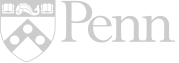## Exactly Measure it – Then Convert it!

Author: Cynthia Campisi

School/Organization:

Francis Scott Key Elementary

Year: 2020

School Subject(s): Math

The unit begins reviewing standard measurement and the vocabulary associated with measurement.  From that point, instruction will focus on new content for converting one type of standard measure to another.  Students will learn how to correctly convert utilizing multiplication and/or division, as well as conversion charts.  Once mastery of this concept is complete, the instruction will continue into measurement with fractional portions.  This concept will focus on measuring to quarter inch sections only.  The unit will conclude with multi step word problems, students will be given direct instruction which include strategies to assist with applying all of the concepts learned throughout this unit.  Throughout this unit, each lesson provides an exit ticket that will offer a demonstration of learning for every individual student.  These exit tickets should be used as a summative assessment and will drive instruction accordingly.   The unit culminates with a test/quiz as a formative assessment for the concepts taught.(2 votes, average: 3.00 out of 5)Loading...

##### Full Unit Text
###### Content Objective:

Over the last 5 years, for fourth grade, in my district, the Pennsylvania State Standard Assessment (PSSA) data for mathematics has steadily declined. The data shows a large learning gap in measurement, especially measurement that involves fractional portions. For several years, this same area of instruction has also proven difficult for students within my own fourth grade classroom.  The lack of conceptual understanding has prompted me into further study as to where the misconceptions of measurement are occurring.  I began to research outside the school district and state to identify if this situation is occurring in other classrooms around the country.  I located an article from the New York Times dated October 30, 2015, whose author, Liana Heitin, describes in great detail the decline throughout the United States in standardized test scores for math and reading.  Examining the data within this article I learned that one of the larger areas in the math decline is around measurement.  In his book, “Measurement in Early and Elementary Education”, Douglas Clements states, “measurement knowledge and strategies play broadly and deeply into children’s understanding of both science and mathematics.” In Chapter one of the book it also states the necessity of working through the elements of theoretical foundation in measurement. As fractions are a vital part of all types of measuring situations, it is imperative that students are able to clearly understand and be able to apply this concept to everyday tasks. It’s not only important in length measurement, but in liquid, weight, and volume measurement as well.

I went back and studied the scope and sequence of our school district requirements as to the grade level and concepts of measurement which must be taught. Students are taught fractions beginning in grade 1; however, the use of fractional portions in a real-world situation does not occur until third grade. At this point, the required instruction in the scope and sequence is limited to: placing fractions on a number line by quarters, comparing unit fractions and ordering fractions with like denominators. Therefore, students are entering fourth grade with limited knowledge and application of fractional portions as it relates to measurement. Considering the lack of conceptual understanding, which would provide a formatted baseline to be built upon, instead, is a concept that needs to be re-taught and expanded upon. A lack of basic mathematical understanding, compounded with the shortage of resource materials in our district, adds to the challenge of teaching the concept of measurement with fractional portions.

Over the last 18 years of teaching mathematics, I have noted that students demonstrate large gaps in their understanding of number sense.  This prompted me to seek alternative teaching methods beyond what my district provides in resource materials and professional development opportunities.  It is important to me, as an educator, to make sure I teach to all the modalities and ensure my students are obtaining the knowledge needed for success.  Therefore, I needed to find resources and professional development seminars that I could utilized in assisting my students and create a structure of knowledge which will enhance understanding and provide them with the ability to apply concepts.  To assist in achieving this goal and extended my knowledge, I became a fellow at the University of Pennsylvania Teachers Institute of Philadelphia as a participant in the course visualization of mathematics.  The course is based on designing and implementing many different forms of visual components to assist students in gaining a better understanding of why and how a concept is applied.   Instruction which includes a visual component will provide students another way to absorb knowledge.

Another method that includes a visual component is exploring using manipulatives or a hands-on method. This is an additional way a student can visualize how numbers work together, giving a window into the true number sense needed to formulate a sum or answer for an equation.  This also provides students an additional strategy as they approach the idea that numbers are more than just numbers.  Students need to visualize why numbers are in a specific place, then manipulating those numbers within those place value positions, to show equivalency.  Students also need to realize that the four mathematical operations are interchangeable (i.e.:  addition and multiplication, subtraction, and division).  Teaching through visualization gives students the opportunity to see exactly why and how these interchanges occur.

There are several objectives for this unit.  The first objective will include students having an increased knowledge of number sense.  The next objective stated is students will be able to utilize knowledge of vocabulary and conversion equivalents, in order to solve problems involving measurement and conversion of measurements from a larger unit to a smaller unit and smaller unit to larger unit.  Then, students will be able to convert standard measurement in a larger unit in terms of a smaller unit, to record measurement equivalents in a two-column table.  Finally, students will be able to use four operations to solve word problems involving distance, involving simple fractions and problems that require expressing measurements given in a larger unit in terms of a smaller unit, in order to represent measurement quantities using diagrams such as number line diagrams that feature a measurement scale.

###### Teaching Strategies:

There are four; ninety-minute lessons in this unit. All the lessons are equipped with small group instruction for those students who need additional reinforcement. Several suggested methods to assess that students are developing learning will include, teacher observation, an exit ticket for the end of each lesson, finally a culmination of all concepts learned will be assessed with a formal test.

In this unit, I will be focusing on the use of visualization as a guide to assist students in envisioning how and why, the parts of a whole work in conjunction with one another. To accomplish this task, I will take into consideration the learning styles, language barriers, and learning disabilities in which students may exhibit. To enhance the student’s interest and willingness to participate in their learning, I will be utilizing read aloud books, group interactive lessons, power point presentations, and manipulatives/rulers to meet the needs of the multi-intelligent styles.

This unit will focus on teaching students several strategies involving fractional measurement beginning with the complex vocabulary utilized in measurement and fractional equations. The lessons will include standard measurement, vocabulary, fractional portions, and conversion of measurement. Included in this unit are auditory, visual, and concert variations to enhance students understanding also giving students the ability to apply concepts when solving real-world equations. Incorporated in this unit are lessons where students will listen to a read aloud story, “How Big is a Foot?” which deals with a measurement conundrum. They will experience visualization through power point presentations, which will demonstrate how fractional portions are part on one whole.  The process of how conversion of measure is linked within inches to feet, and feet to yards, etc, will also be demonstrated through the visual medium of power point presentations. The creation of anchor charts, as an additional resource for students to utilize, will include vocabulary, fractional portions of a ruler, and conversion equations.  These charts will assist student when completing assignments.  The use of manipulatives will be integrated as students work with their small groups assisting one another with the task of measuring.

To implement this unit, the materials suggested are: (1) chart paper to create vocabulary anchor chart, and a chart showing conversion equations; (2) next, a ruler copied onto oak-tag, or stock paper, along with colored pencils — these will be utilized through out the unit.  Then, (3) a twelve-inch ruler for each student and several yard sticks for each group.  In addition, (4) index cards can be used to create exit tickets for each lesson.  Finally, (5) students will need some type of composition book to make notes and solve equations.  There are several worksheets in the appendix of this unit that can be utilized with each lesson.

###### Classroom Activities:

Lesson One Powerpoint

Meaurement to fractional portions Powerpoint

Lesson One addresses the common core standard 4.MD.A, the use of a read aloud “How Big is a Foot?” will be used to activate background knowledge about measurement. To continue the process of background a vocabulary anchor chart will be created.  This chart will include, both the entire word, as well as, the standard abbreviation for each word.  These charts should be placed in a convenient location as a future student resource.  Next, the students will have a hands-on opportunity to maneuver one-inch sections along the twelve-inch ruler.  Then, students will work in groups using one another’s ruler to prove how three twelve-inch rulers make one yard.  These activities are to prepare students to understand the conversion from inches to feet and feet to yards.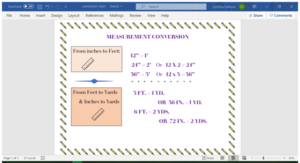The exit ticket for this lesson will offer insight into the students’ understanding or misconception of the conversion method.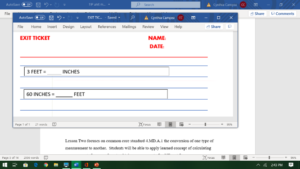Lesson Two focuses on common core standard 4.MD.A.1 the conversion of one type of measurement to another.  Students will be able to apply learned concept of calculating measurements from one form to obtain measurement of a different form.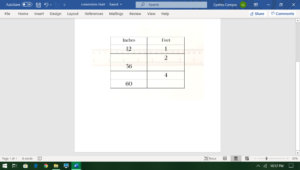To assess student knowledge of this concept, pass out an index card with a pre-printed conversion chart for student to complete.  The exit ticket should require students to show their work!

The focus for Lesson three is the common core standard 4.MD.A.2 which requires students to measure using fractions parts.  The classroom activity to meet this standard is to have students color coordinate their paper rulers.  The use of color coordinating fractions as specific points on the paper ruler will give students a focal point to relay upon for standard measurement.  Along with this activity, each group will be given sets of fraction bars to manipulate in guided practice.  To assist students with where and how to locate fraction parts on the ruler a power point presentation is provided.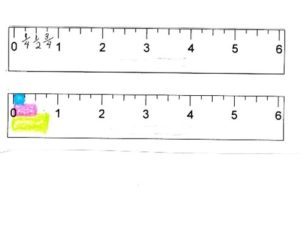The exit ticket for this lesson will be to have students draw a line first; then they will need to measure the line and plot the points of the measurement.  Finally, they will need to give the exact total measurement of their line.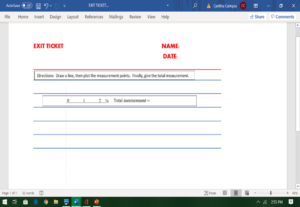Lesson four teaches students the standard 4.MD.A.2 which wants students to use the four operations to solve word problems involving distances.  To achieve this objective a student must have prior knowledge involving addition, subtraction, multiplication, and division.  It is also recommended that students have a good understanding surrounding the concept of how to obtain information within the word problem to solve it correctly.  To begin place a word problem on the board and read the problem all the way through with the entire class.  Then go back and slowly re-read the problem as you circle or highlight the numbers needed and underline the question.  Next, have students discuss the mathematic operations they would use to solve this equation.  Have a student volunteer come to the board and solve the equations, explaining each step in the process.Then, have the students work either in groups or independently to solve the next word problem.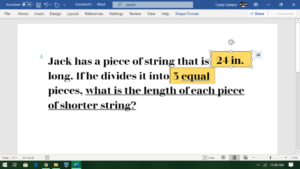Once you observed most of the students understanding of the concept, have them complete the worksheet and conduct small group instruction as needed.  At the conclusion of the lesson review answers to the worksheet and have students complete an exit to check for understanding.Upon review of all exit tickets, decide if most of the students have a sufficient knowledge base to formally assess.  For lesson five, review the concepts taught in this unit, so that the students are demonstrating the ability to apply the conceptual knowledge of the unit, administer the quiz/test.

Lesson Plan – Day 1

Title: Standard Measurement

Length:  90 minutes (45 minute lesson, 45 minutes for stations and small group instruction)

Common Core Standard: 4.MD.A

Objective:  Students will be able to utilize knowledge of vocabulary and conversion equivalents to solve problems involving measurement and conversion of measurements from a larger unit to a smaller unit and smaller unit to larger unit.

Materials:

Vocabulary anchor chart

Conversion chart-standard measurement

Ruler, Yard Stick, paper sections of inch, feet.

Composition books

Paper ruler – inch, feet, yard (worksheet #1)

Procedures:

Beginning

1. Read aloud – “How Big is a Foot”
2. Introduce standard measurement vocabulary; inch, feet, yard, mile, acre, include

Abbreviations (in., ft., yd., mi., ac.)( “ inch, ‘ foot)

1. Create anchor chart with students for future reference of standard measurement

vocabulary.

During

1. Demonstrate, using paper 1” sections and 1-12” ruler, to visually equate the measurement

of 12-1” section as equivalent to 12” or 1 foot.  (Worksheet #1)

1. Model, (through power point presentation (1)) using rulers and yard stick, to visually show

students the connections between 3-

12” rulers and 1-36” yard stick.

1. Using paper sections, work with students in a guided practice of converting inches to a

foot and feet to a yard. (worksheet #1)

1. Model how to use addition and/or multiplication to begin larger conversions.

ie;  24 inches = ____2___ feet,  3 feet = ____36___inches,  1 yard = ________feet

1 foot = 12 inches                    If 1 foot is 12” then

1 foot = 12 inches                     3’ x 12” = 36”

2 feet = 24 inches

1. Provide students with additional conversion practice problems. (Worksheet #2)

After

1. Ask if students have any questions or concerns.
2. Students will complete exit ticket as a demonstration of learning/understanding

Small group instruction – differentiation

1. Students will work along with teacher using paper sections to assist with conversion.
2. Students will work together assisting one another as they problem solve conversions,

teacher will observe and intervene when necessary.

Lesson Plan – Day 2

Title:  Standard Measurement conversion table

Length:  90 minutes  (45 minute lesson, 45 minutes for stations and small group instruction)

Common Core Standard:  4.MD.A.1

Objective:  Students will be able to convert standard measurement in a larger unit in terms of a smaller unit in order to record measurement equivalents in a two-column table.

Materials:

Two-column chart

Conversion chart (worksheet #2)

Conversion practice (worksheet #3)

Index cards

Procedures:

Beginning

1. Review standard measurement vocabulary
2. Review steps to make standard measurement conversions.

During

1. Create a two-column conversion chart with the entire class.
2. Model for students the step-by-step process of converting from one measurement unit to

another.

 Feet Inches 2 24 3 36 4 48 5 60 6 72

1. Given specific measurement set in which students will create a two-column conversion

chart. (student chart will convert yards and feet)

After

1. Review chart, whole class, check for student understanding or misconceptions.
2. Distribute index cards and ask student to complete the conversion chart.

Small group instructions – differentiation

1. Teacher will work with students modeling the correct process of converting one

measurement to another.  To enhance understanding teacher may utilize inch markers, 12” ruler, yard stick.

1. Students will work along with teacher converting measurement.

Lesson Plan – Day 3

Title:  Standard measurement using fractional portions

Length:  90 minutes (45 minute lesson, 45 minutes for stations and small group instruction)

Common Core Standard:  4.MD.A.2

Objective: Students will be able to use four operations to solve word problems involving

distance, involving simple fractions and problems that require expressing measurements given in a larger unit in terms of a smaller unit. In order to represent measurement quantities using diagrams such as number line diagrams that feature a measurement scale.

Materials:

Paper rulers – (Worksheet #4)

Color pencils

Index cards

Measuring with fraction portion – (Worksheet #5)

Procedures:

Beginning

1. Review standard measurement vocabulary
2. Activate background knowledge regarding fractional portions.

model fractional portions, ¼, ½, ¾, (students will use fraction bars to enhance

understanding.)

1. Explain that all measured items are not always measured to a whole number, many times

they are only a fraction of the whole number.

During

1. Demonstrate, using power point, where fractional portions are located on a ruler.
2.   Distribute paper rulers and color pencils
3. Guide students along the power point presentation(2) as they color code their rulers into

fractions portions.

1. Students will now have an opportunity to practice using paper rulers to measure lines on

(worksheet #4) *emphasis the whole number of the measurement must be included.

After

1. Review worksheet, whole class, check for student understanding or misconceptions.
2. Exit Ticket:  Distribute index cards and ask student to create a line using a selected

measurement of their choosing, students must draw the line and indicate the position of

the whole numbers, then, write the complete measurement of the line, which must

include a fractional part.

ie; ___________________

0             1             2    ¼     Total measurement = 2 ¼ “

Small group instructions – differentiation

1. Teacher will work with students modeling the correct process of measuring, (student may

need a reminder/anchor chart, as to how to; start at the end of the line/item, and at the                     end of the ruler or 0, where the line/item stops, look at the number on the ruler at the end

of the item/line and this the measurement.

1. Students will work along with teacher practicing the measuring of the line/item.

Lesson Plan – Day 4

Title:  Standard measurement – word problems

Length:  90 minutes (45 minute lesson, 45 minutes for stations and small group instruction)

Common Core Standard:  4.MD.A.2

Objective: Students will be able to use four operations to solve word problems involving

distance, involving simple fractions and problems that require expressing measurements given in a larger unit in terms of a smaller unit. In order to represent measurement quantities using diagrams such as number line diagrams that feature a measurement scale.

Materials:

Word Problem (Worksheet #6)

Procedures:

Beginning

1. Review standard measurement vocabulary.
2. Activate background knowledge regarding adding and subtracting fraction.

3   Review conversion of standard measurement

During

1. Describe the steps needed to complete word problems.
2. Read the entire word problem
3. Underline the question
4. Circle the numbers needed to solve the equation
5. Choose the correct mathematical operation(s) need to solve the equation
6. Display word problem, go through each of the steps above.  Once all information is

outlined begin mathematical operation(s) and solve the equation.

1. Display a second word problem for students to work in guided practice.
2. Once students display understanding of this concept, supply independent practice.

After

1. Upon completion of independent and small group work, come back as a whole class to

review independent work.  Consider having students work in small groups reviewing

each other’s work, circling incorrect answers.  Give students the opportunity to re-do

work before working through the equations with them to provide the correct answer.

1. Exit Ticket:  Jamie’s jump rope measures 28 inches, and Ashley’s jump rope measures                                 Who has the larger jump rope?  Show your work:

Small group instruction – differentiation

1. Students will work with teacher, reading, reviewing, underling, circling information within the word problem.
2. Teacher will prompt students to assist with finding correct information and choosing the proper mathematic operation(s) to solve the equation.

Lesson – Day 5

Title:  Standard Measurement conversion table

Length:  90 minutes (45 minutes for quiz, 45 minutes for stations and/or game)

Common Core Standard:  4.MD.A, 4.MD.A.1, 4.MD.A.2

Materials:

Conversion Quiz (Worksheet #7)

Before

1. Review previously learned concepts learned during this unit.

During

1. Administer quiz to formally assess knowledge of concept.

After

1. Ask if students have any questions regarding the equations on the quiz.
2. Clarify any misconceptions.

###### Resources:

Articles:

Heitin, L., “Drop in U.S. Math, Reading Scores Prompts Blame Game”, New York Times, 2015, 30, October.

Books:

Clements, D., Barrett, J., Sarama, J. “Measurement in Early and Elementary Education”, chapter 1; 2011.

Myller, R., “How Big is a Foot?”, Yearling, 1991.

Worksheets:

“Convert between Yards, Feet and Inches – K5 Learning.” K5Learning,     www.k5learning.com/worksheets/math/grade-4-converting-units-length-yards-feet-inches-f.pdf.

Length Word Problems (Feet & Inches) – K5 Learning.” K5Learning, www.k5learning.com/worksheets/math/grade-4-length-word-problems-a.pdf.

“COVERTING YARDS, FEET AND INCHES 2.” Math-Salamanders, math-salamanders.s3-us-west-1.amazonaws.com/Measurement/Converting-Measures/Converting-Customary-Units/converting-yards-feet-inches-2.pdf.

See PDF for worksheets

References:

Pearson Education, 2014, 2015, 2016, 2017, 2018, Pennsylvania Department of Education, Pennsylvania State Standard Assessment. education.pa.gov/DataAndReporting/Assessments/Pages/PSSA-Results.aspx

###### Appendix:

Standards:  (Common Core)

4.MD.A

Solve problems involving measurement and conversion of measurements from a larger unit to a smaller unit.

4.MD.A.1

Know relative sizes of measurement units within one system of units including km, m, cm; kg, g; lb, oz.; l, ml; hr, min, sec. Within a single system of measurement, express measurements in a larger unit in terms of a smaller unit. Record measurement equivalents in a two-column table. For example, know that 1 ft is 12 times as long as 1 in. Express the length of a 4 ft snake as 48 in. Generate a conversion able for feet and inches listing the number pairs (1, 12), (2, 24),

4.MD.A.2

Use the four operations to solve word problems involving distances, intervals of time, liquid volumes, masses of objects, and money, including problems involving simple fractions or decimals, and problems that require expressing measurements given in a larger unit in terms of a

smaller unit. Represent measurement quantities using diagrams such as number line diagrams that feature a measurement scale.